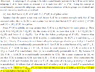# Chief factor of finite group

moont14263
How did the author get that all the elements of order p or 4 of L are contained in K? I mentioned the abstract but I do not think there is a need for that.

Help?

#### Attachments

•Untitled20.png
50.3 KB · Views: 473

Take $x\in L$. Then there is a g in G such that $x\in T^g$ and thus there is a y in T such that $x=gyg^{-1}$. If x has order p or order 4, then so does y. But by the previous sentence, y is contained in K. Since K is normal, we have that also x is in K.
The symbol $L=\bigcup_{g \in G} T^{g}$, does it mean the union of sets or $L=<T^{g},g \in G>$and, if it the union of sets, then how did he gets that $L$ equals to that union?